Tavakkoli, A., Hemmasi, A. H., Talaeipour, M., Bazyar, B., and Tajdini, A. (2015). "Forecasting of particleboard consumption in Iran using univariate time series models," BioRes. 10(2), 2032-2043.

#### Abstract

The performance of the Autoregressive Integrated Moving Average )ARIMA) model and Double and Holt-winters exponential smoothing techniques for forecasting the consumption of particleboard in Iran are compared. Annual time series data from 1978 to 2009 in the modeling process, and observations from 2010 to 2012 were used to check the accuracy of the models’ forecasting performance. Also, the models’ performances were calculated in terms of RMSE criterion, and the consumption of particleboard in Iran was forecasted up to the year 2017 using the most appropriate model. The results of comparing different forecast models showed that the ARMA (2,1) model yielded the lowest RMSE value compared to the other two models, which makes it more appropriate for the prediction of consumption of particleboard in Iran. Results also revealed that there might be an increasing trend in the consumption of particleboard, i.e., an average annual increasing rate calculated as 5% for particleboard. Thus, it was predicted that the consumption of particleboard would increase from 901,652 m3 in 2012 to 1,178,320 m3 in 2017.

Forecasting of Particleboard Consumption in Iran Using Univariate Time Series Models

Amir Tavakkoli,a Amir Hooman Hemmasi,a,* Mohammad Talaeipour,a Behzad Bazyar,a and Ajang Tajdini b

The performance of the Autoregressive Integrated Moving Average )ARIMA) model and Double and Holt-winters exponential smoothing techniques for forecasting the consumption of particleboard in Iran are compared. Annual time series data from 1978 to 2009 in the modeling process, and observations from 2010 to 2012 were used to check the accuracy of the models’ forecasting performance. Also, the models’ performances were calculated in terms of RMSE criterion, and the consumption of particleboard in Iran was forecasted up to the year 2017 using the most appropriate model. The results of comparing different forecast models showed that the ARMA (2,1) model yielded the lowest RMSE value compared to the other two models, which makes it more appropriate for the prediction of consumption of particleboard in Iran. Results also revealed that there might be an increasing trend in the consumption of particleboard, i.e., an average annual increasing rate calculated as 5% for particleboard. Thus, it was predicted that the consumption of particleboard would increase from 901,652 m3 in 2012 to 1,178,320 m3 in 2017.

Keywords: Forecasting; Particleboard consumption; Double exponential smoothing; Holt-Winters exponential smoothing; ARIMA

Contact information: a: Department of Wood and Paper Science, Tehran Science and Research Branch, Islamic Azad University, Tehran, Iran; b: Department of Wood and Paper Science, Karaj Branch, Islamic Azad University, Karaj, Iran; *Corresponding author: h_hemmasi@yahoo.com

INTRODUCTION

Forecasts are produced in a wide range of fields, as they are important tools for decision making. In this regard, forecasting of forest products’ demand is one of the key inputs for successful market planning in the forest sector (Hetemaki and Mikkola 2005). Among different forest products, wood-based panels, such as particleboard, have enjoyed an extremely wide range of applications in furniture and joinery as well as in the building and construction industries (Tajdini et al. 2014).

The particleboard industry in Iran is one of the oldest industries in the wood-based panels industry section. It has lasted more than 50 years, and in comparison with other wood-based panels, such as fiberboard, plywood, and veneer, it has a relatively favorable economic situation at the moment. In 2012, there were more than 19 active manufacturing factories with a total nominal capacity of 1,078,890 m3, which produced 881,634 m3 of particleboard (85% of the total nominal capacity). Therefore, with respect to 901,652 m3 consumption for this product in 2012, it can be stated that 97.8% of the total consumption of the country was produced by the local manufacturers and the remainder was supplied through importation (Ministry of Industries and Mines of Iran 2013). The available data also showed that over 32,001 m3 of particleboard, with a price of \$8 million, was imported in 2012. In the same year, the amount of export was 11,983 m(Tehran Chamber of Commerce, Industries, Mines and Agriculture 2013).

The particleboard industry has the potential to use wood wastes including branches, cotton and corn surplus, flax and hemp, and other woody plants. Conversely, the particleboard industry is considered to be able to satisfy the demand of raw woody materials, which reduces the pressure on the Iranian forests. Therefore, because of the importance of this product for different purposes, such as manufacturing furniture and other applications, the prediction of particleboard future demand would be helpful from different aspects. Several methods have been applied to forecast in forest products markets in various parts of world. For instance, the lumber demand in the United States was forecasted by Alexander et al. (2003) using time series analysis methods. Song (2006) compared and predicated the price of softwood lumber in US using univariate forecasting methods (ARIMA, Spectral, simple Lag and simple dummy-variable models), as well as multi-equation models (VAR, 2SLS and EMC models). Bayatkashkoli et al. (2008) forecasted the export and import rates of wood and wooden products, such as particleboard and plywood in Iran, using forecasting techniques of trend and regression formulations. Arabatzis and Klonaris (2009) analyzed the Greek aggregate import demand for unprocessed wood (such as logs), processed wood (such as sawn wood), veneer crafts (such as veneer sheets) and wood manufactures during the period of 1969 to 2001 using the linear approximation of quadratic AIDS (QUAIDS) model. Azizi et al. (2009) provided projection for consumption of particleboard, fiberboard, MDF, and plywood in Iran using the exponential smoothing model until 2012. Ioannou et al. (2009) used the Artificial Neural Networks (ANN) model to predict the prices of conifer fuel wood in Greece. Koutroumanidis et al. (2009) used the ARIMA and Artificial Neural Networks (ANN) models as well as a hybrid approach (ARIMA-ANN) to predict the future selling prices of the fuel wood (from broadleaved and coniferous species) produced by Greek state forest farms. Emang et al. (2010) used univariate time series models, including Holt-winters-seasonal, ARAR algorithm, and seasonal ARIMA modeling to forecast the future volume of exporting wooden products (including chipboard and moulding) in Malaysia. Mohammadi Limaei et al. (2011) analyzed time series and an autoregressive procedure to predict the export and import of wood in Iran. Tajdini et al. (2014) forecasted the consumption of wood-based panels in Iran using exponential smoothing and ARIMA models until 2014.

This study investigates the predictive power of univariate time series models include: Double exponential smoothing model, Holt-winters exponential smoothing model, and Autoregressive Integrated Moving Average (ARIMA), as well as the consumption of particleboard in Iran, was forecasted for the period of 2013-2017 using the most appropriate model. Conveying information regarding the trend of coming consumption enables policy makers to develop long-term programs for the manufacturing sector and formulate appropriate strategies so as to meet the domestic demand.

EXPERIMENTAL

Methodology

Univariate time series models

The time series models frequently consider the variable’s behavior in the past as the basis for forecasting. Before using these models, it is necessary to survey their randomness, because if the data are random, it would not be possible to use forecasting models based on the past trend. To do this, the parametric test of Durbin-Watson and the non-parametric test of Runs were used. Also, to perform the Durbin-Watson test, it is necessary that the observations conform to a normal distribution. In this study, the observations’ normality was investigated using the Kolmogorov-Smirnov (KS) test.

Exponential smoothing (ES) models

Exponential smoothing is a procedure used for the continuous revision of a forecast in light of more recent experience. This method is a relatively simple, yet robust approach to forecasting. It is widely used in business for forecasting demand for inventories (Gardner 1985). It has also performed surprisingly well in forecasting competitions against more sophisticated approaches (Makridakis and Hibon 2000).

Single exponential smoothing (SES) model

SES model is a procedure that repeats enumeration continually using the most recent data. This method can be used if the data is not significantly influenced by trend and seasonal factors. The single exponential smoothing model for a time series (yt) is given by the model equation: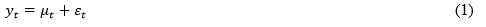The smoothing equation is: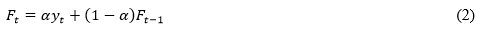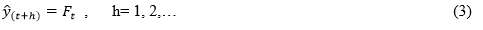where urepresents the time-varying mean(level) term ,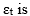a white noise error termand a is a smoothing constant between 0 and 1. The smoothed statistic Ft is a simple weighted average of the previous observation and the previous smoothed statistic (Ft−1).

Double exponential smoothing (DES) model

The DES model is a refinement of the popular single exponential smoothing model that includes another component, which takes into account any trend in the data. When, there is an increasing or decreasing trend in the data over time, single exponential smoothing forecasts tend to lag behind observations. Double exponential smoothing is designed to deal with such data by taking their trends into account. The double exponential smoothing model for a time series (yt) is given by the model equation: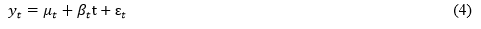The smoothing equations are,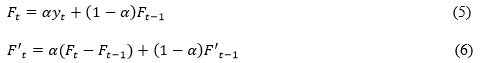where urepresents the time-varying mean(level) term,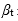represents the time varying slope term (called “trend” term by exponential smoothers) ɛt is a white noise error term, Ft is the single smoothed series, and F’t is the double smoothed series. The symbol α stands for the smoothing parameter, between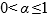.The forecasts from double smoothing are computed using the following formula.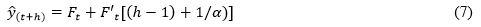Holt-Winters exponential smoothing (HWES) model

HWES model is similar to the double method, but it uses parameters that are different from the one used in the original series to smooth the trend value. The prediction of Holt-Winters exponential smoothing can be obtained by using two smoothing constants and the following three equations,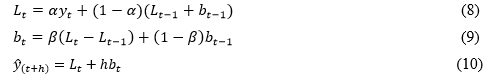where yt is the observed value at time tLt is the forecast at time tbt is the estimated slope at time tais the first smoothing constant used to smooth the observations,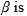the second smoothing constant used to smooth the trend, and yt + h is forecast value for period t + h . It must be mentioned that and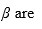determined by minimizing the sum of squares of the forecast errors (MSE or RMSE).

Autoregressive integrated moving average (ARIMA) model

In this method, which is also called the Box-Jenkins (BJ) method, the behavior of the dependent variable (y) is explained using its values in the past and stochastic error terms (Box and Jenkins 1978). This method includes a series of four steps (Gujarati 2004): (1) Model Selection, where the order of autoregressive (p) and moving average (q) are determined after recognizing the variable’s stationary degree (d). To do this, maximum lag orders of three (which have the minimum Akaike Information Criterion (AIC)), are used to select the values of p and q in the ARIMA (pdq) process (Pesaran and Pesaran 1997). Using this criterion in small samples requires special attention, without which the forecasting uncertainty may increase. Therefore, the RMSE criterion is also used for assessing the forecasting accuracy (Marcellinio et al. 2006), so that the forecasting error (in terms of RMSE) would be minimized for the minimum Akaike criterion. The following equation is used for AIC computation.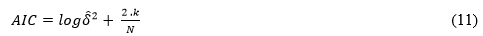where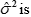the estimated variance of white noise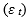, N is the number of observations and k is the number of estimated parameters, e.g., k = p + q.

(2) Model estimation is followed by (3) model checking. This is where normality and autocorrelation of model residuals are tested. If the residuals have a normal distribution, and their autocorrelation and partial correlation coefficients (ACFs and PACFs) are not significant among lags, it would be possible to accept the selective model as a desirable fit (Newaz 2008). Otherwise, it should be rejected and the previous stages should be repeated. (4) Forecasting, where the selected and estimated model is typically simulated forward to produce forecasts for the variable of interests at one or more relevant horizons.

The ARIMA procedure is performed on stationary data. Stationary is necessary in this method because only then one can legitimately learn from the past to predict the future realization of a time series. Otherwise, the density of the observations estimated from the past data will not be helpful in predicting future observations. This study considers the stationary of series by two unit root tests of Augmented Dickey-Fuller (ADF) and Philips-Perron(PP). In Box and Jenkins’ notation, a process is called ARIMA(p,d,q), if it yields a stationary ARMA(p,q) process after differencing it, d times. In practice, only the cases d =0,1,2 are used, where d=0 indicates a stationary ARMA process. In other words, ARIMA models get reduced to ARMA models; thus, ARIMA(p, 0, q)= ARMA(p,q), (Gujarati 2004). The general form of an ARMA model is as follows,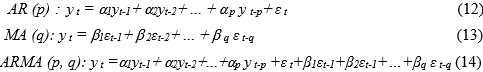where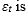white noise, identically normally distributed with zero mean and variance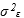Evaluation Measures of Model Fitting

In this study, the demand of particleboard is determined based on an apparent consumption method (Apparent Consumption= Production + Import – Export). As a result, forecasts using consumption models reflect the apparent consumption estimate (Gupta et al. 2013). The complete data set must be dividing into two parts, fitting the model to the first part, but keeping back the second part, called the test set, so that predictions from the model can be compared with the observed values (Chatfield 2001). There is no principle for this dividing. In accordance with using annual data and their properties from observation limits views as well as improving forecasting performance as a result of increasing required data for model estimation, about 90% of the data (from 1978-2009) were used for estimating or fitting the forecasting models, and the remaining 10% (from 2010-2012) were used for the out-of-sample forecast performance evaluation (models validation).

Finally, forecasting was performed for the period of 2013-2017, applying the appropriate model. Also, the forecasts were evaluated by analyzing the pattern of forecasts relative to the actual values using the RMSE criterion, which is the most commonly used measure for comparing relative accuracy of forecasting models (Clements and Hendry 1998). In forecast evaluation, the use of RMSE is also justified, as it is a familiar and easily interpretable measure; the lower the RMSE, the better the forecast (Malaty 2007) and RMSE values less than 0.1 indicate good model fit (Pindyck and Rubinfeld 1998). The RMSE is found using the following equation,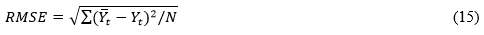where Yt is the observed value,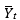is the forecasted value, and N is the number of forecast periods.

Throughout all the forecasting procedures, the data in Napier logarithm scale were used in the models and thus the predicted values were in logarithmic form as well. After performing all the calculations, these variables were returned into their first condition for the purpose of comparing and interpreting the results. The Eviews 7 and SPSS software packages were utilized for all necessary data processing and calculations.

RESULTS AND DISCUSSION

Univariate Time Series Models

In these models, it is necessary to check the stationarity of series. Stationary means that the mean and variance of the series are constant and also the covariance is time-invariant (Gujarati 2004). The results obtained from the stationary of particleboard consumption are displayed in Table 1.

Table 1. Results of the ADF and PP Unit Root Tests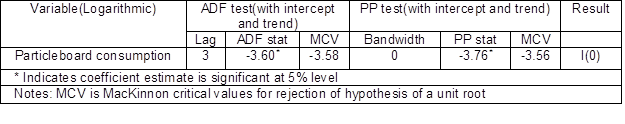The results of the unit root tests indicated that the null hypothesis, the unit root is in series, is rejected using the ADF and PP tests at 5% critical value. Therefore, the particleboard consumption series is integrated of order zero, or I(0). Also, the results of the normality and randomness tests of series, which are necessary for forecasting with time series models, are presented in Tables 2, 3, and 4.

Table 2. Results of the Kolmogorov-Smirnov Normality Test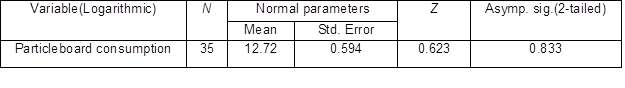Table 3. Results of the Parametric Durbin-Watson Test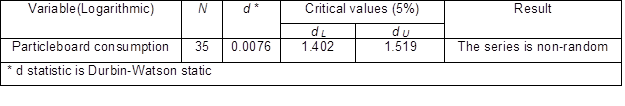Table 4. Results of the Non-Parametric Runs Test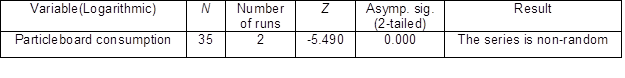The results of the series normality test indicate that the p-value of the series is not significant at the 5% level (Table 2). As a result, the null hypothesis that the time series have a normal distribution cannot be rejected. Therefore, the particleboard consumption series can be assumed to have a normal distribution, meaning that it is possible to use the parametric test of Durbin-Watson for investigating the series randomness. As shown in Table 3, the results of this test indicate that the null hypothesis, based on the lack of positive autocorrelation for particleboard consumption series, is rejected (0<d<dL). Therefore, the variable is non-random and predictable. Also, the results of the Runs test severely reject the null hypothesis, showing that the variable is random at 5% level. It also emphasizes the non-random and predictable nature of this series (Table 4).

Exponential smoothing (ES) models

The results of particleboard consumption forecasting using Double and Holt-Winters exponential smoothing techniques are displayed in Table 5.

Table 5. Results of Particleboard Consumption Forecasting (in m3) Using Exponential Smoothing (ES) Models, 2010-2012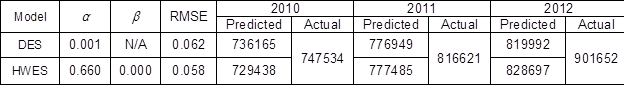The final values of the smoothing coefficients for Double and Holt exponential smoothing models are shown in Table 5. The parameters with the smallest RMSE were used to forecast. As shown in the Table 5, the values predicted using both models are lower than the actual (observed) values for 2010 to 2012. Also, the value of RMSE for the Holt-Winters exponential smoothing model (0.058) is less than the one for the Double exponential smoothing model (0.062). This indicates that the model fitting degree for both Double and Holt-Winters exponential smoothing models is good (Pindyck and Rubinfeld 1998), but, the forecasting accuracy of the Holt-Winters exponential smoothing model is higher than the Double exponential smoothing model; thus, it is more desirable for forecasting.

ARIMA model

When modeling with ARIMA, it is necessary to have a stationary time series (Gujarati 2004; Kunst 2011). According to the results shown in Table 1, this goal is achieved at the 5% level. Therefore, in ARIMA (pdq), d = 0, and the ARMA (pq) model can be used for forecasting (Gujarati 2004). In this study, the trend (in addition to the constant) was taken into consideration in the model. If the trend was significant in the model, its effect will be omitted from the model, otherwise, modeling will be performed without it. The results of model selection are displayed in Tables 6 and 7.

Table 6. RMSE and AIC Values Based on Different Orders of p and q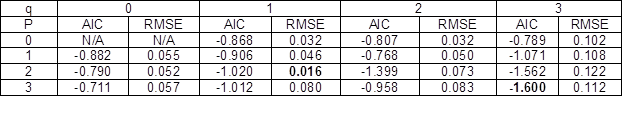Table 7. Comparison of ARMA(2,1) and ARMA(3,3) Models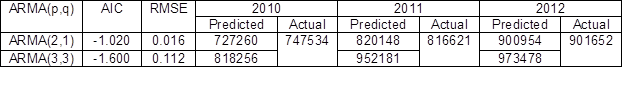In this study, the approaches given by Pesaran and Pesaran (1997) and Marcellino et al. (2006) are used to determine the autoregressive (p) and moving average (q) orders. As shown in Table 6, the results of recognizing an ARMA (pq) model indicated that ARMA (3,3), based on the minimum AIC, and ARMA (2,1), based on the minimum RMSE, are the best models for forecasting particleboard consumption in Iran. Therefore, both models were estimated and the series’ forecasted values were compared with the actual ones. Finally, ARMA (2,1) was selected as the final model, because the difference between the Predicted and the actual values was lower for 2010- 2012, as shown in Table 7. The results of estimation using this model and the NLS method, as well as the results of checking the adequacy of the ARMA (2,1) model, are indicated in Tables 8 and 9.

Table 8. Estimation Results of ARMA (2,1) Model with Constant and Trend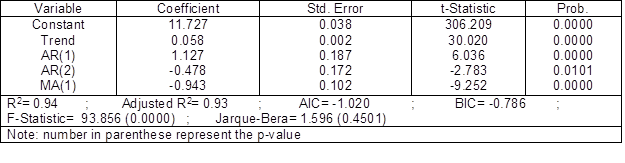Table 9. Correlogram Results for the Residual of the ARMA (2,1) Model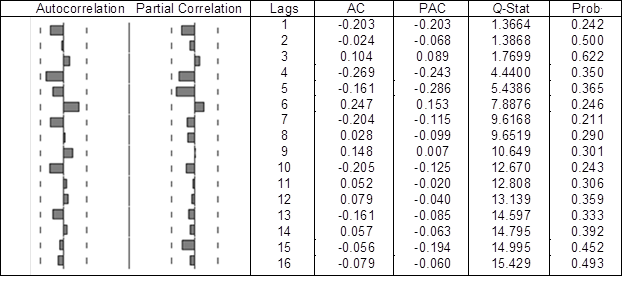As shown in Table 8, all of the model’s parameters are statistically significant at the 5% level (p-value ˂ 0.05). The coefficient of determination (0.94) and the F-statistic (93.9) indicate that the model is valid. Also, to investigate the residuals’ normality, the Jarque-Bera test was performed, which indicates that they are normally distributed. As shown in the correlogram in Table 9, none of the autocorrelation (ACFs) and partial correlation (PACFs) coefficients of the residuals were found to be statistically significant at the 5% level. Overall, the residuals of the ARMA (2,1) model are completely random and follow a white noise process (Newaz 2008).Therefore, the chosen model is desirable for forecasting, and there is no need to search for another one. The results of particleboard consumption forecasting using the ARMA (2,1) model is displayed in Table 10.

Table 10. Results of Particleboard Consumption Forecasting Using ARMA (2,1) Model (in m3)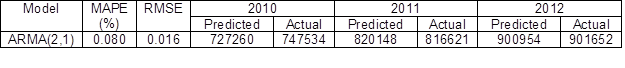According to the results of forecasting accuracy evaluation of the ARMA (2,1) model for 2010 to 2012, which are shown in Table 10, the forecasted values for particleboard consumption series are almost equal to the actual (observed) values for 2010 to 2012. Also, the RMSE is 0.016 and the percentage of prediction error (MAPE) is less than 1%. This indicates that, the model fitting degree is good (Pindyck and Rubinfeld 1998), Thus, this prediction is excellent.

Forecast Comparison and Evaluation

The results of comparing different forecast models are displayed in Table 11.

Table 11. Results of Accuracy Evaluation of the Different Forecast Models for Particleboard Consumption (in m3)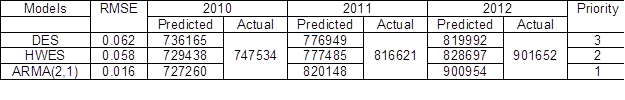As shown in Table 11, the ARMA (2,1) model has the lowest RMSE (0.016) and, since the RMSE value is less than 0.1, this indicates that the model fitting is good (Pindyck and Rubinfeld 1998). Also, the forecasted values for particleboard consumption series are almost equal to the actual (observed) values for the period of 2010 to 2012, as the ARMA(2,1) model forecasting range was estimated from 727,260 m3 in 2010 to 900,954 m3 in 2012, while its actual values were in the range of 747,534 m3 to 901,652 m3. Therefore, in terms of prediction accuracy, it is proposed that ARMA is the best among these models and the Holt-Winters and Double exponential smoothing models stand in the second and third positions, respectively. Therefore, the following ARMA model is used to forecast particleboard consumption in 2013-2017 as displayed in Table 8.

yt =11.73 + 0.06Trend + 1.13yt-1 – 0.48yt-2 – 0.94ɛt-1      (16)

The above model was specified by the highest R2 and adjusted Rvalues (0.94 and 0.93, respectively), and the smallest RMSE value (0.016). The arithmetic coefficients were significant (p-value ˂ 0.05), there was normality of residuals, and residuals of the model could be regarded as white noise (there is no significant in the ACFs and PACFs of the residuals) )Newas 2008).

Table 12. Forecasted Values of Particleboard Consumption in Iran over the Period 2013-2017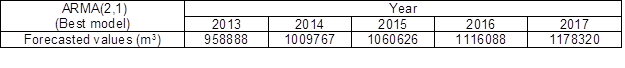According to the study of Azizi et al. (2009) and Tajdini et al. (2014), there is an increasing trend in the consumption of particleboard in Iran and it can be expected to have an average annual growth rate of 5% over the forecast period. This means that it is can increase by 30.7% from 901,652 m3 in 2012 to 1,178,320 m3 in 2017 (Table 12).

CONCLUSIONS

1. The results of both the parametric test of Durbin-Watson and non-parametric test of Runs for investigating the series randomness indicate that the randomness hypothesis of series can be rejected, meaning that particleboard consumption series is non-random and predictable and it is possible to use forecasting models based on the past trend.
2. In this study the accuracies of univariate time series models were compared for forecasting the consumption of particleboard in Iran. Results showed that all univariate time series models (DES, HWES, and ARMA models) are fully suitable models for forecasting of particleboard consumption in Iran (they have the RMSE value less than 0.1), but, since the ARMA model has higher forecasting accuracy than the exponential smoothing models, therefore, it is more appropriate for the five years forecast of particleboard consumption in Iran.
3. The results of forecasting by using ARMA model revealed that the consumption of particleboard in Iran, despite strong substitutes such as MDF, is forecasted to increase by an average of 5% per year, from 901,652 m3 in 2012 to 1,178,320 m3 in 2017.

ACKNOWLEDGMENTS

We want to thank the three anonymous referees, the associate editor, and the editor M. B. Parsi for their very useful comments and suggestions on an earlier version of our paper. All errors remain our own.

REFERENCES CITED

Azizi, M., Ghorbanzadeh, H., and Hatefnia, H. (2009). “Estimation of demand for wood panels in Iran by the year 2012,” J. For. Res. 20(1), 179-182. DOI: 10.1007/s11676-009-0033-z

Bayatkashkoli, A., Rafeghi, A., Azizi, M., Amiri, S., and Kabourani, A. (2008). “Estimate of timber and wood products export and import trend in Iran,” J. Agric. Sci. Nat. Resour. 15(1), 73-83.

Box, G. P. E., and Jenkins, G. M. (1978). Time Series Analysis: Forecasting and Control, Holden Day, San Francisco.

Chatfield, C. (2001). Time-Series Forecasting, Chapman & Hall/CRC, London.

Clements, M., and Hendry, D. (1998). Forecasting Economic Time Series, Cambridge University Press, Cambridge.

Emang, D., Shitan, M., Ghani, A. N. A., and Noor, K. M. (2010). “Forecasting with univariate time series models: A case of export demand for peninsular Malaysia’s moulding and chipboard,” J. Sust. Dev. 3(3), 157-161. DOI: 10.5539/jsd.v3n3p157

Gardner, E. S. (1985). “Exponential smoothing: The state of the art,” J. Forecast. 4(1), 1-28. DOI: 10.1002/for.3980040103

Gujarati, D. N. (2004). Basic Econometrics, 4th (ed.), McGraw Hill, New York.

Gupta, M., Corrie, K., Hug, B., and Burns, K. (2013). “Preliminary long-term forecasts of wood product demand in Australia,” Australian Government, Department of Agriculture, Fisheries and Forestry, Canberra.

Hetemaki, L., and Mikkola, J. (2005). “Forecasting Germany’s printing and writing paper imports,” For. Sci. 51(5), 483-493.

Arabatzis, G., and Klonaris, S. (2009). “An analysis of Greek wood and wood product imports: Evidence from the linear quadratic Almost Ideal Demand System (AIDS),” For. Policy Econ. 11(4), 266-270. DOI:10.1016/j.forpol.2009.04.002

Ioannou, K., Arabatzis, G., and Lefakis, P. (2009). “Predicting the prices of forest energy resources with the use of Artificial Neural Networks (ANNs): The case of conifer fuel wood in Greece,” JEnviron. ProtEcol. 10(3), 678-694.

Koutroumanidis, T., Ioannou, K., and Arabatzis, G. (2009). “Predicting fuelwood prices in Greece with the use of ARIMA models, artificial neural networks and a hybrid ARIMA-ΑΝΝ model,” Energy Policy 37(9), 3627-3634.

Kunst, R. M. (2011). Applied Time Series Analysis- Part ΙΙ, University of Vienna and Institute for Advanced Studies Vienna.

Makridakis, S., and Hibon, M. (2000). “The M3-Competition: Results, conclusions and implications,” Int. J. Forecast. 16(4), 451-476. DOI:10.1016/S0169-2070(00)00057-1

Malaty, R., Toppinen, A., and Vitanen, J. (2007). “Modeling and forecasting Finnish pine sawlog stumpage prices using alternative time-series methods,” Can. J. For. Res. 37(7), 178-187. DOI: 10.1139/X06-208

Marcellinio, M., Stock, J. H., and Watson, M. W. (2006). “A comparison of direct and indirect and iterated multi step AR methods for forecasting macroeconomic time series,” J. Econometrics 135(1-2), 499-526. DOI: 10.1016/j.jeconom.2005.07.020

Ministry of Industries and Mines of Iran (2013). Available at: http://www.mimt.gov.ir. Accessed: June 15, 2013.

Mohammadi Limaei, S., Heybatian, R., Heshmatol Vaezin, S. M., and Torkman, J. (2011). “Wood import and export and its relation to major macroeconomics variables in Iran,” For. Policy Econ. 13(4), 303-307. DOI: 10.1016/j.forpol.2011.03.001

Newaz, M. K. (2008). “Comparing the performance of time series models for forecasting exchange rate,” BRACU J. 5(2), 55-65.

Pesaran, H. M., and Pesaran, B. (1997). Working with Microfit 4.0: An Introduction to Econometrics, Oxford University Press, Oxford.

Pindyck, R.S., and Rubinfeld, D.L. (1998). Econometric Models and Economic Forecasts, 4th (ed.), McGraw-Hill, New York.

Song, N. (2006). Structural and Forecasting Softwood Lumber Models with a Time Series Approach, Ph.D. dissertation, Louisiana State University, Baton Rouge, LA.

Tajdini, A., Tavakkoli, A., Latibari, A. J., Roohnia, M., and Pourmousa, S. (2014). “Statistical modeling to forecast the wood-based panels consumption in Iran,” Int. J. Biosci. 4(12), 1-11. DOI: 10.12692/jib/4.12.1-11

Tehran Chamber of Commerce, Industries, Mines and Agriculture (2013). Available at: http://www.tccim.ir. Accessed: July 6, 2013.

Article submitted: October 31, 2014; Peer review completed: January 11, 2015; Revised version received and accepted: February 2, 2015; Published: February 4, 2015.

DOI: 10.15376/biores.10.2.2032-2043